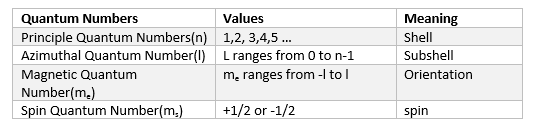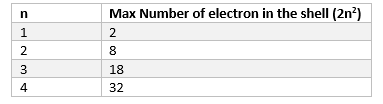Home » Chemistry » How to Find Quantum Numbers for an atom

# How to Find Quantum Numbers for an atom

There are four Quantum Numbers for the each electron in the atom. In this post we will see how to find Quantum Numbers for the electrons in an atom or elements .

In order to find the Quantum numbers, we need to consider two things.

a. Quantum number range and meaning

b.How the electrons are distributed in various order in the atom.

Brief Introduction about Quantum Numbers and Range

There are four Quantum numbers

a. Principle Quantum Number

b. Azimuthal Quantum  Number

c. Magnetic Quantum number

d.Spin Quantum number

The first three are the solutions of Schrodinger equation

Range of values defined for each of them is given asThe maximum numbers of electrons in shell  is given byArrangement of electrons in  the atom

Electrons are filled up in the atom as per the three rules

1. Aufbau rule or Principle which states that in ground state of atom, the orbitals are filled in increasing order of their energies.
The sum of the values of the principal quantum numbers (n) and azimuthal quantum number (l) i.e (n+l) determined the energy level of an orbital.

Based on above, the electron are filled in below order of the orbitals

1s, 2s, 2p, 3s, 3p, 4s, 3d, 4p, 5s, 4d, 5p, 4f,5d, 6p, 7s

2. Pauli exclusion Principle which states no two electrons can have same set of 4 quantum no.
It can be also stated as only two electron can exists in the orbital and these electrons must have opposite spin

3. Hund ’s rule of maximum multiplicity which states that pairing of electrons in the orbital’s belonging to same sub – shell [ p, d, f], does not take place until each orbital gets singly occupied.

Quantum numbers together with above three rules defined the configuration of the electron in the  atom.

We need to perform the below steps to find quantum numbers for the each electrons in the atom

1. Write the Electronic configuration of the atom as per the rules given above
2. Once the configuration is determined , we can easily write the four quantum numbers for each of the electrons in the atoms

So lets take some example to understand it better

Hydrogen ( Atomic Number -1)

In Hydrogen, there is one electron only, so it will be in First orbit

1s

Quantum Numbers for the electron will be

n=1 , l=0 , m=0, s=1/2

Helium ( Atomic Number -2)

In Helium, there is two electron only, so it will be in First orbit

1s2

Quantum Numbers for the electron will be

First electron

n=1 , l=0 , m=0, s=1/2

Second electron

n=1 , l=0 , m=0, s=-1/2

Carbon(Atomic number 6)

There are 6 electrons in the carbon atom which means 2 electron to be placed in first shell and 4 electrons in the second shell

So electronic configuration will be

1s2 , 2s2 , 2p2

Quantum Numbers for the electron will be

First electron

n=1 , l=0 , m=0, s=1/2

Second electron

n=1 , l=0 , m=0, s=1/2

Third electron

n=2 , l=0 , m=0, s=1/2

Fourth  electron

n=2 , l=0 , m=0, s=-1/2

Fifth  electron

n=2 , l=1 , m=-1, s=1/2

Sixth  electron

n=2 , l=1 , m=0, s=1/2

Sodium (Z=11)

So electronic configuration will be

1s2 , 2s2 , 2p6,3s1

First electron

n=1,l= 0,m= 0,s= ½

Second electron
n=1,l= 0,m= 0 ,s=-½

Third electron

n=2,l= 0, m=0,s= ½

Fourth electron
n=2,l= 0,m= 0,s= -½

Fifth electron

n=2,l= 1,m= -1,s= ½

Sixth electron
n=2,l= 1,m= 0,s= ½

Seventh electron
n=2, l=1,m= 1,s= ½

Eighth electron
n=2,l= 1,m= -1,s= -½

Ninth electron
n=2, l=1,m= 0,s= -½

Tenth electron
n=2,l= 1,m= 1,s= -½

Eleventh electron

n=3,l= 0,m= 0,s= ½

### 1 thought on “How to Find Quantum Numbers for an atom”

1. Thanks for adding me into this WhatsApp group

This site uses Akismet to reduce spam. Learn how your comment data is processed.